Courses

# Concepts of Convective Heat Transfer Chemical Engineering Notes | EduRev

## Chemical Engineering : Concepts of Convective Heat Transfer Chemical Engineering Notes | EduRev

The document Concepts of Convective Heat Transfer Chemical Engineering Notes | EduRev is a part of the Chemical Engineering Course Heat Transfer.
All you need of Chemical Engineering at this link: Chemical Engineering

Convective Heat Transfer Examples

1. Melt Spinning of Polymer fibers

2. Heat transfer in a Condenser

3. Temperature control of a Re-entry vehicle

Fiber spinning

The fiber spinning process presents a unique engineering problem, primarily due to the effects of shape variations, heat and possibly the viscoelastic behavior of the materials (polymers for example) typically used. This becomes evident when the design of the spinneret geometry is needed to produce a specified fiber size and shape. Determining the proper die geometry given the desired final fiber shape is further complicated by the heat and viscoelastic effects. In addition, since the fiber is pulled from the spinneret, the final dimensions of the fiber are difficult to determine. The effects of viscous heating and air cooling must be monitored to ensure that the material does not degrade because of extreme local temperatures, often difficult to measure because of the small size. The stresses and deformation of the material must also be predicted to avoid the fiber from breaking. All these effects complicate the design of the fiber spinning process.

Definition of a Heat Transfer Coefficient

For heat transfer in conducting systems, we have seen that we can express the heat flux across a surface S as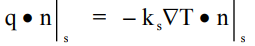We have used a similar representation to develop detailed descriptions of mass and transfer in a number of situations where the physics or chemistry is well-understood, e.g., permeation through a membrane, heat transfer to a sphere. We showed that we could get a description of the macroscopic transfer across an interface by the use of a Heat Transfer Coefficient.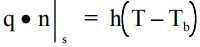We do nor always have such a good model or understanding. The are other equivalent physical situations, e.g., turbulent flow in a pipe. There we use a measure of the frictional loss in the pipe as a “momentum transfer coefficient”. The dimensionless form was the Friction Factor. The dimensionless mass transfer coefficient is the Nusselt Number.

Nu = hL/k

Methods of Analysis

1. Detailed Solution of the Conservation Laws

2. Approximate Analysis

3. Dimensional Analysis

4. Empirical Correlation of Data

We have seen several examples of Detailed Solution and we have done some Approximate Analysis, e.g., mass transfer to or from a flowing film, heat transfer from a solid sphere. What we did was to transform the exact problem into a simpler more solvable one using mathematical analysis. What we do in the next few lectures is examine in greater detail the last three methods as tools to analyze heat transfer and to design processes.

Approximate Analysis and Film Theory

Film Theory is the simplest and oldest approach in the use of mass transfer coefficients and in their prediction. The Theory is attributed to Nernst.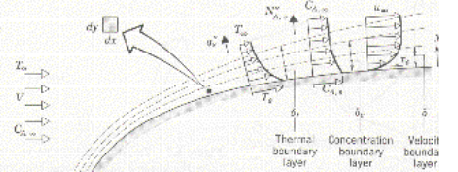Examine the neighborhood of the phase boundary. We assume that the flow field consists of two regions, a uniform region in the bulk of the fluid far from the surface and a region in the vicinity of the boundary where viscosity dominates (since there is no slip at the boundary).

Film Model

The film model presumes that the velocity field is linearized in some sense near the boundary so that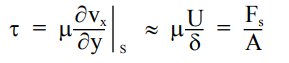This means that the film has a thickness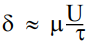But recall the definition of the friction factor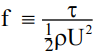If we introduce that notion into our analysis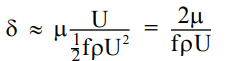Not surprisingly we can relate the fractional layer thickness to the Reynolds number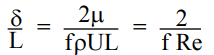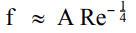Dimensionless Heat Transfer Coefficient

We defined the Heat Transfer Coefficient, h , by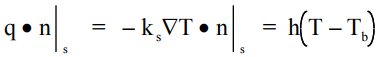so that the Nusselt number is given as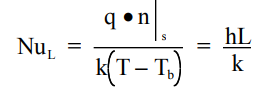Observe that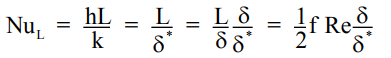so that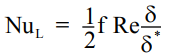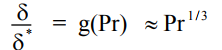Then we observe a relation rather like the ones we calculated in our more detailed models.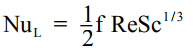The Chilton-Colburn Analogy

In the 1930s, based on the Nernst Film Theory, two duPont researchers proposed an analogy between heat transfer (and we have seen, mass transfer) and momentum transfer. They defined a dimensionless number termed a j-factor, jH.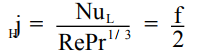For mass transfer, the relation was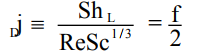Simple film theory, then, predicts that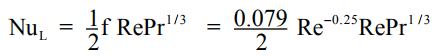or simplified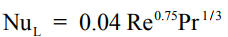Offer running on EduRev: Apply code STAYHOME200 to get INR 200 off on our premium plan EduRev Infinity!

## Heat Transfer

59 videos|70 docs|85 tests

,

,

,

,

,

,

,

,

,

,

,

,

,

,

,

,

,

,

,

,

,

;